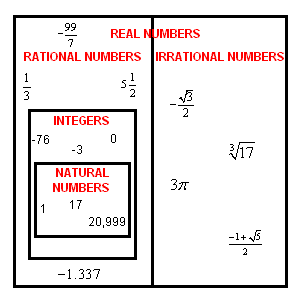The rational numbers are those numbers which can be expressed as a ratio between two integers. For example, the fractions $\frac{1}{3}$ and $-\frac{1111}{8}$ are both rational numbers. All the integers are included in the rational numbers, since any integer $z$ can be written as the ratio $\frac{z}{1}$ .

Numbers which cannot be written as a ratio of integers are called irrational .

All decimals which terminate are rational numbers (since $8.27$ can be written as $\frac{827}{100}$ .) Decimals which have a repeating pattern after some point are also rationals: for example,

$0.0\text{83333333}\dots =\frac{1}{12}$ .

The set of rational numbers is closed under all four basic operations: that is, given any two rational numbers, their sum, difference, product, and quotient is also a rational number (as long as we don't divide by $0$ .)

The Venn diagram below shows the relationships of the various sets of numbers.